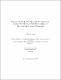## Second-order least squares estimation in regression models with application to measurement error problems##### Date
2009-01-21T15:17:56Z
Abarin, Taraneh
##### Abstract
This thesis studies the Second-order Least Squares (SLS) estimation method in regression models with and without measurement error. Applications of the methodology in general quasi-likelihood and variance function models, censored models, and linear and generalized linear models are examined and strong consistency and asymptotic normality are established. To overcome the numerical difficulties of minimizing an objective function that involves multiple integrals, a simulation-based SLS estimator is used and its asymptotic properties are studied. Finite sample performances of the estimators in all of the studied models are investigated through simulation studies.
##### Keywords
Nonlinear regression, Censored regression model, Generalized linear models, Measurement error, Consistency, Asymptotic normality, Least squares method, Method of moments, Heterogeneity, Instrumental variable, Simulation-based estimation
##### Citation
Abarin, T. and Wang, L. (2009). Second-Order Least Squares Estimation of Censored Regression Models. J. STAT. Plan. Infer. 139, 125-135.
Abarin, T. and Wang, L. (2006). Comparison of GMM with Second-order Least Squares Estimation in Nonlinear Models. Far East J. Theo. Stat. 20 (2), 179 -- 196.